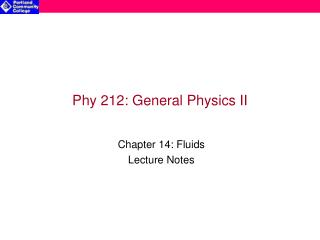# Phy 212: General Physics II - PowerPoint PPT PresentationDownload PresentationPhy 212: General Physics II

Phy 212: General Physics II
Download Presentation## Phy 212: General Physics II

- - - - - - - - - - - - - - - - - - - - - - - - - - - E N D - - - - - - - - - - - - - - - - - - - - - - - - - - -
##### Presentation Transcript

1. Phy 212: General Physics II Chapter 14: Fluids Lecture Notes

2. Daniel Bernoulli (1700-1782) • Swiss merchant, doctor & mathematician • Worked on: • Vibrating strings • Ocean tides • Kinetic theory • Demonstrated that as the velocity of a fluid increases its pressure decreases (known as Bernoulli’s Principle)

3. What are fluids? Fluids are substances that continually deform (flow) under an applied shear stress regardless of how small the applied stress. All liquids and all gases are fluids. Useful Physical Quantities: • Mass Density (r) is mass per unit volume or The SI units of mass density are kg/m3 • Pressure is the magnitude of the normal force (DFN) applied to a surface per unit area (DA), or The SI units of pressure are newtons/meters2 (N/m2) or pascals (Pa) Notes: • For static fluids (at rest), force can only be applied normal to the surface • Tangential (or shear) forces result in fluid flow!

4. Pressure in Fluids • Fluids are mobile • Fluids do not retain their shape • Fluids do not independently support their shape • The pressure within a fluid varies with depth • Pressure within a fluid is constant at all points of the same depth • Pressure increases with increasing depth (H) P ~ H {where H is the depth below the surface of the fluid} • The pressure difference (DP) between 2 points within a fluid is related to the density of the fluid (r) & the depth difference between the points • The greater the fluid density (r), the greater DP • The greater the depth difference (DH), the greater DP P2 – P1 = rgDH

5. F1=P1A A DH mg F2=P2A Derivation of pressures in fluids Consider a disk of water in a static fluid: • Applying Newton’s 2nd Law to the disk: or • The mass of the disk is:m = rADH so Newton’s 2nd Law can be rewritten as P2A – P1A = mg = (rADH)g or P2 – P1 = rgDH • Note that pressure in a fluid does not depend on: • The total mass of the fluid disk • The surface area where the force is applied

6. Atmospheric Pressure • If the surface of a fluid is exposed to air, the pressure at that surface (Po) is equal to that of the atmospheric air pressure at that elevation, this is a consequence of Pascal’s Principle. • At sea level the atmospheric pressure is Po = 1.01 x 105 N/m2 • The pressure at any depth (H) below the surface of the fluid is given by: P = Po + rgH • This relationship also applies to air pressure at higher altitudes (to the extent that the air density does not change substantially)

7. How high can you suck water up using a straw? (or pumping water uphill) In order to draw water (or any fluid) upward you must lower the pressure difference between the pressure inside the “straw” and the outside environment (usually the atmosphere). 1. Is there a limit to how low you can make the pressure inside a straw? 2. How high can you suck water?

8. Barometers Barometers are devices used to measure air (or atmospheric) pressure • Types of barometers: Closed end Open end

9. Pascal’s Principle When pressure is applied to an enclosed fluid, the pressure is transmitted undiminished to all parts of the fluid and the enclosing walls • The effect of applied pressure is to increase the Po for the fluid. • The application of hydraulics is based on Pascal’s Principle: • P2 = P1 + rgH • or • Therefore

10. Archimedes’ Principle When an object is submerged in a fluid, the fluid exerts a buoyant force (FB) on the object equal to the weight of the fluid displaced by the object: FB = mfluidg = rfluidVdisplacedg Notes: • When a submerged object has greater density than the fluid, its weight will be greater than FB (it will sink) • When a submerged object has lower density than the fluid, it will partially submerge in the fluid until it reaches a depth where its weight will be the same as FB (it will float) • When the submerged object has the same density as the fluid it will neither sink nor float but will remain in equilibrium as long as it is completely submerged

11. Equation of Continuity When a fluid flows, the rate of mass entering a point must be equal to the rate of mass exiting the point: Or (since & ) r1A1v1 = r2A2v2 Where: • A is the cross-sectional area of the fluid segment • Dx is the width of the fluid segment

12. Bernoulli’s Equation Bernoulli’s Principle: as speed of fluid increases its pressure drops Bernoulli’s principle can be extended to Bernoulli’s equation for a (ideal) fluid that is: • Nonviscous (no friction or interaction with sidewalls) • Incompressible (liquid does not expand nor compress) Bernoulli’s Equation: P1 + rgy1 + ½ rv12 = P2 + rgy2 + ½ rv22 Which can be rewritten as P2 - P1 = - rgy1 - ½ rv12 + rgy2 + ½ rv22 Or: P2 - P1 = rg(y1 - y2) + ½ r(v12 -v22) Note: the “state” of the fluid is constant at all points: P1 + rgy1 + ½ rv12 = P2 + rgy2 + ½ rv22 = constant